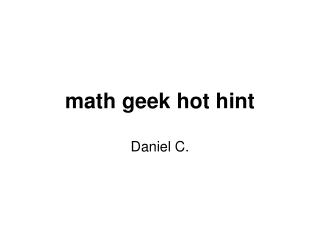DownloadDownload Presentationmath geek hot hint

math geek hot hint

Télécharger la présentationmath geek hot hint

- - - - - - - - - - - - - - - - - - - - - - - - - - - E N D - - - - - - - - - - - - - - - - - - - - - - - - - - -
Presentation Transcript

1. math geek hot hint Daniel C.

2. Hot Hints To decimal You divide the top number by the bottom number 3/5=0.6 Converting a fraction 3/5 To percentage To make a decimal to a percent you move the decimal to the right two times.

3. Hot Hints To a decimal To make a percent to adecimal you move the decimal point to the left twice. 50%= .50 Converting a Percent 50% To a fraction

4. Hot Hints Converting a Decimal Add decimal To percentage Add steps To a fraction Add steps